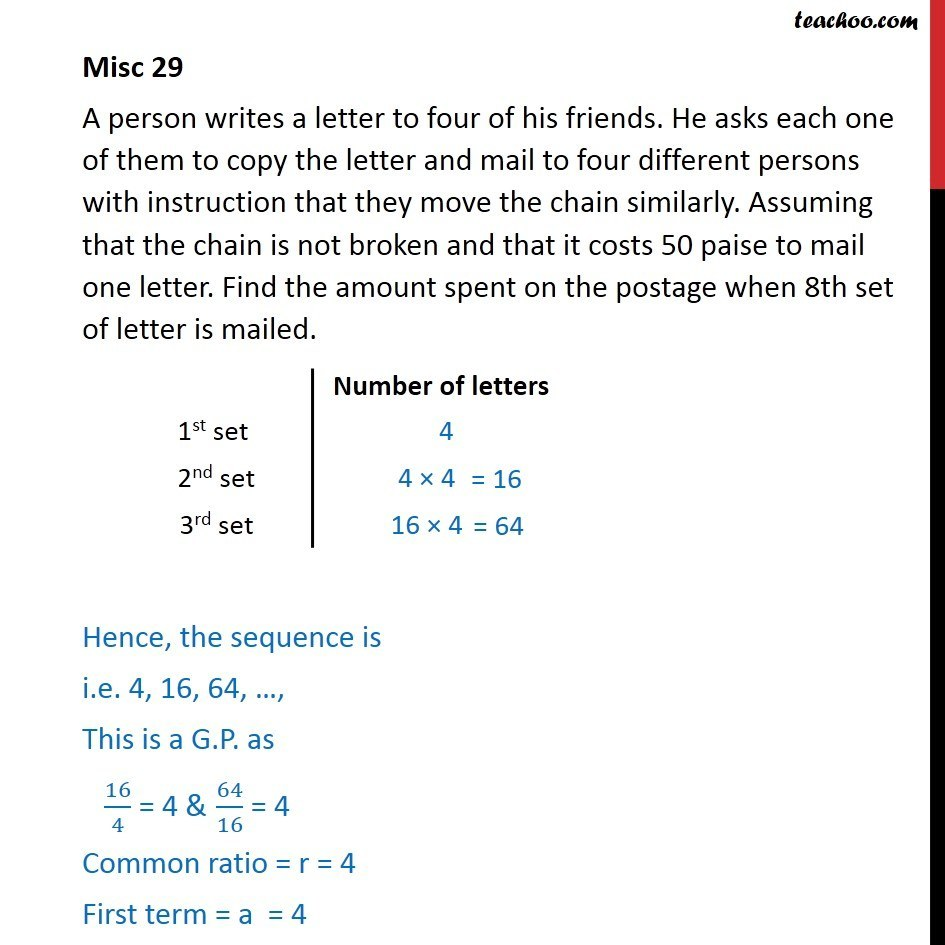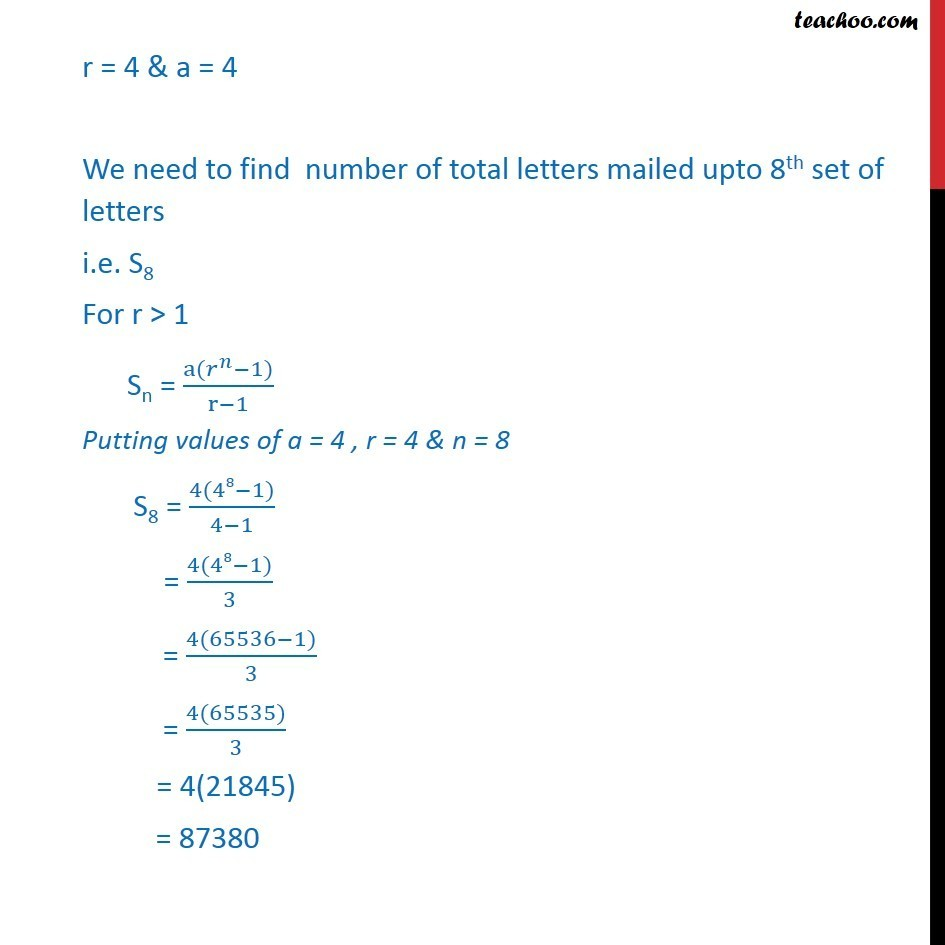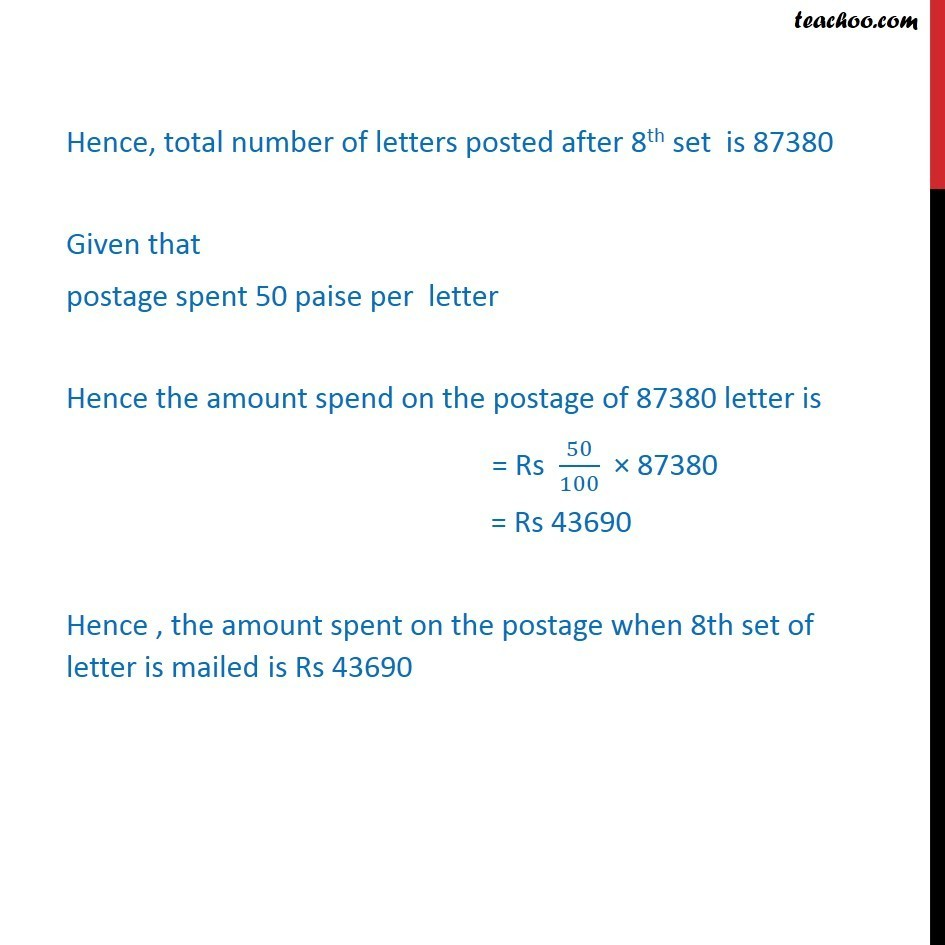Geometric Progression(GP): Statement

Chapter 9 Class 11 Sequences and Series (Term 1)
Concept wise### Transcript

Misc 29 A person writes a letter to four of his friends. He asks each one of them to copy the letter and mail to four different persons with instruction that they move the chain similarly. Assuming that the chain is not broken and that it costs 50 paise to mail one letter. Find the amount spent on the postage when 8th set of letter is mailed. Hence, the sequence is i.e. 4, 16, 64, , This is a G.P. as 16/4 = 4 & 64/16 = 4 Common ratio = r = 4 First term = a = 4 r = 4 & a = 4 We need to find number of total letters mailed upto 8th set of letters i.e. S8 For r > 1 Sn = (a( ^ 1))/(r 1) Putting values of a = 4 , r = 4 & n = 8 S8 = (4(48 1))/(4 1) = (4(48 1))/3 = (4(65536 1))/3 = (4(65535))/3 = 4(21845) = 87380 r = 4 & a = 4 We need to find number of total letters mailed upto 8th set of letters i.e. S8 For r > 1 Sn = (a( ^ 1))/(r 1) Putting values of a = 4 , r = 4 & n = 8 S8 = (4(48 1))/(4 1) = (4(48 1))/3 = (4(65536 1))/3 = (4(65535))/3 = 4(21845) = 87380 r = 4 & a = 4 We need to find number of total letters mailed upto 8th set of letters i.e. S8 For r > 1 Sn = (a( ^ 1))/(r 1) Putting values of a = 4 , r = 4 & n = 8 S8 = (4(48 1))/(4 1) = (4(48 1))/3 = (4(65536 1))/3 = (4(65535))/3 = 4(21845) = 87380 r = 4 & a = 4 We need to find number of total letters mailed upto 8th set of letters i.e. S8 For r > 1 Sn = (a( ^ 1))/(r 1) Putting values of a = 4 , r = 4 & n = 8 S8 = (4(48 1))/(4 1) = (4(48 1))/3 = (4(65536 1))/3 = (4(65535))/3 = 4(21845) = 87380 r = 4 & a = 4 We need to find number of total letters mailed upto 8th set of letters i.e. S8 For r > 1 Sn = (a( ^ 1))/(r 1) Putting values of a = 4 , r = 4 & n = 8 S8 = (4(48 1))/(4 1) = (4(48 1))/3 = (4(65536 1))/3 = (4(65535))/3 = 4(21845) = 87380 r = 4 & a = 4 We need to find number of total letters mailed upto 8th set of letters i.e. S8 For r > 1 Sn = (a( ^ 1))/(r 1) Putting values of a = 4 , r = 4 & n = 8 S8 = (4(48 1))/(4 1) = (4(48 1))/3 = (4(65536 1))/3 = (4(65535))/3 = 4(21845) = 87380 r = 4 & a = 4 We need to find number of total letters mailed upto 8th set of letters i.e. S8 For r > 1 Sn = (a( ^ 1))/(r 1) Putting values of a = 4 , r = 4 & n = 8 S8 = (4(48 1))/(4 1) = (4(48 1))/3 = (4(65536 1))/3 = (4(65535))/3 = 4(21845) = 87380 r = 4 & a = 4 We need to find number of total letters mailed upto 8th set of letters i.e. S8 For r > 1 Sn = (a( ^ 1))/(r 1) Putting values of a = 4 , r = 4 & n = 8 S8 = (4(48 1))/(4 1) = (4(48 1))/3 = (4(65536 1))/3 = (4(65535))/3 = 4(21845) = 87380 Hence, total number of letters posted after 8th set is 87380 Given that postage spent 50 paise per letter Hence the amount spend on the postage of 87380 letter is = Rs 50/100 87380 = Rs 43690 Hence , the amount spent on the postage when 8th set of letter is mailed is Rs 43690